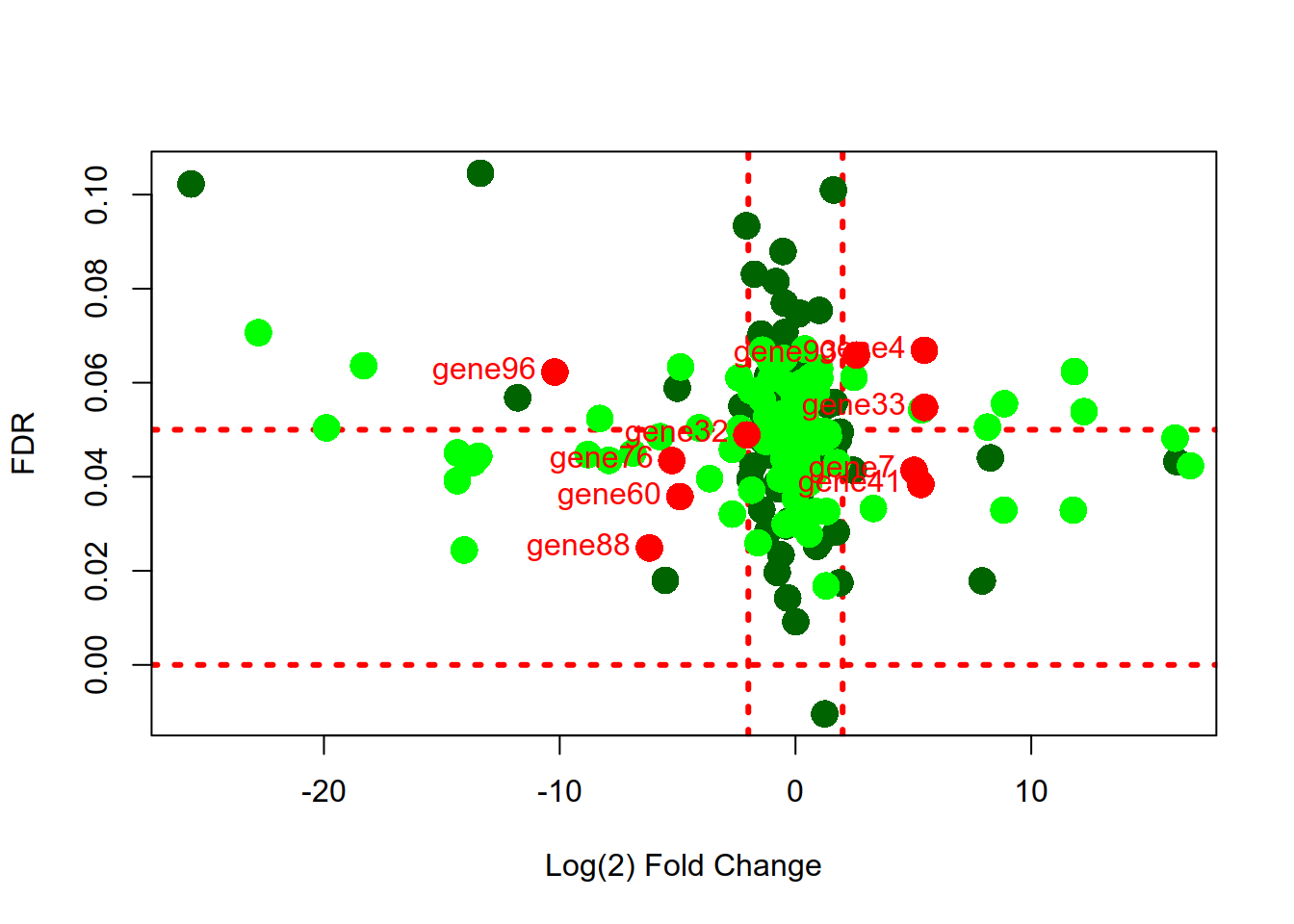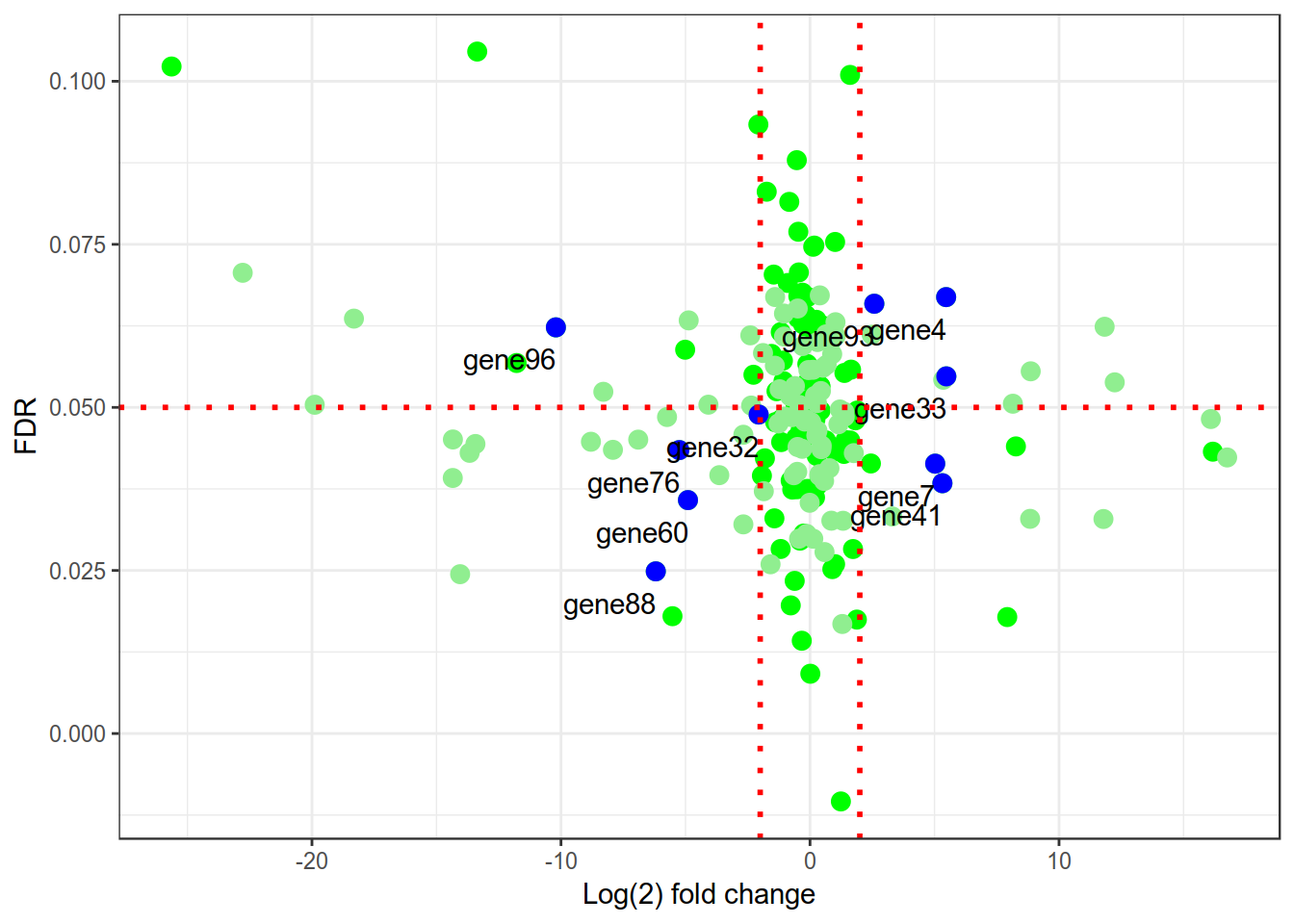### Volcano plot

Volcano plot is not new. In the era of microarrays, they were used in conjunction with MA plots. Volcano plot is a plot between p-values (Adjusted p-values, q-values, -log10P and other transformed p-values) on Y-axis and fold change (mostly log2 transformed fold change values) on X-axis. Then one adds all kinds of decorations to plot like cut-off lines so and so forth. It is really surprising to see that there is no way of plotting volcano plot directly in ggplot2 like barplot considering extensive use of ggplot by bioinformatics scientists. In this note, two data frames will be simulated.Each data frame will have 100 genes with log fold changes and adjusted p-values. Both the dataframes share 10 genes with identical fold changes and p-values. We are going to highlight genes by sample and the common genes will be highlighted in a different color.

### Simulate data for two datasets

#### simulate a data frame “edge”

``````set.seed(100)
edge = data.frame(
gene = paste0("gene",sample(100)),
logFC = c(rnorm(80,0,1),rnorm(20,0,12)),
logFDR = rnorm(100,mean=0.05, sd=0.02)
)``````

#### simulate a data frame “dsq”

``````dsq =data.frame(
gene = paste0("gene",sample(100)),
logFC = c(rnorm(70,0,1),rnorm(30,0,12)),
logFDR = rnorm(100,mean=0.05, sd=0.01)
)``````
Now two data frames (edge and dsq) are simulated, let us create a list of genes that would be shared between both the data frames.
``set.seed(200)``
Create a dataframe by random sampling genes from edge. These will be used in replacing corresponding genes in data frame 2 (dsq) so that these 10 genes are shared between both the data frames.
``cf=edge[abs(edge\$logFC)>2,][sample(nrow(edge[abs(edge\$logFC)>2,]),10),]``
Replace the matching genes from dsq with those from cf
``dsq[dsq\$gene %in% cf\$gene,]=cf``
Sort the data frames by genes
``````edge.sorted=edge[with(edge,order(gene)),]
dsq.sorted=dsq[with(dsq,order(gene)),]``````

### Basic plotting

In this note we will see how to plot expression values vs p-values using basic plotting and ggplot2 in R. #### plot first data frame “edge” Plot would have Log Fold changes on X axis and FDR (Ajusted p-values) on Y-axis. Values would be highlighted in dark green color. Cut offs are drawn in red color.
``````with(edge.sorted,
plot (logFC, logFDR,
col = "darkgreen",
pch = 16,
cex=2,
xlab="Log(2) Fold Change",
ylab="FDR",
abline(v=c(-2,2),h=c(0,0.05), col="red", lty=3,lwd=3)
))

# Now overlay second plot
with (dsq.sorted,
points(
x = logFC,
y = logFDR,
col = "green",
pch = 16,
cex=2
))

# Highlight points of interest
with(edge.sorted,
points(
x = logFC[logFC == dsq.sorted\$logFC],
y = logFDR[logFDR == dsq.sorted\$logFDR],
col = "red",
pch = 16,
cex=2
))

# Add labels to points of interest
with (edge.sorted,
text(
x = logFC[logFC == dsq.sorted\$logFC],
y = logFDR[logFDR == dsq.sorted\$logFDR],
gene[logFC == dsq.sorted\$logFC] ,
cex = 1,
pos=2,
col = "red"
))``````### With ggplot2

``````suppressPackageStartupMessages(library(dplyr))
suppressPackageStartupMessages(library(ggplot2))
suppressPackageStartupMessages(library(ggrepel))``````

#### Data preparation includes merging both the data frames by gene names and then create a second dataframe with common genes. Ofcourse we can reuse cf data frame above.

``````dfm = merge(edge.sorted, dsq.sorted, by = "gene")
dfm1 = dfm[dfm\$logFC.x == dfm\$logFC.y & dfm\$logFDR.x == dfm\$logFDR.y, ]``````

#### Now let us plot the data in ggplot2

``````ggplot(dfm) +
geom_point(
data = dfm,
aes(x = logFC.x, y = logFDR.x),
color = "green",
cex = 3
) +
geom_point(
data = dfm,
aes(x = logFC.y, y = logFDR.y),
color = "lightgreen",
cex = 3
) +
geom_point(
data = dfm1,
aes(x = logFC.x, y = logFDR.x),
color = "blue",
cex = 3
) +
geom_text(
data = dfm1,
aes(x = logFC.x, y = logFDR.x, label = gene),
hjust = 1,
vjust = 2
) +
theme_bw() +
xlab("Log(2) fold change") +
ylab("FDR") +
geom_vline(
xintercept = 2,
col = "red",
linetype = "dotted",
size = 1
) +
geom_vline(
xintercept = -2,
col = "red",
linetype = "dotted",
size = 1
) +
geom_hline(
yintercept = 0.05,
col = "red",
linetype = "dotted",
size = 1
)``````